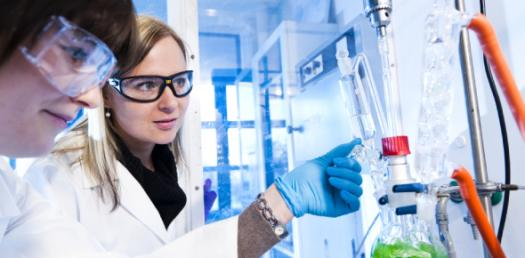# Chemistry: Chemical Reaction Exam Questions

4 QuestionsSettings.

Related Topics
• 1.
A solution containing 20.0g of dissolved sodium hydroxide is added to a solution containing 25.0g of aluminium nitrate.  Which is the limiting reagent?
• A.

NaOH

• B.

Aluminum Nitrate

• C.

Aluminium Hydroxide

• D.

Sodium Nitrate

• 2.
A solution containing 20.0g of dissolved sodium hydroxide is added to a solution containing 25.0g of aluminium nitrate.  What mass of precipitate is formed?
• A.

0.117g

• B.

Cannot be determined

• C.

9.15g

• 3.
40ml of 0.2M HCl is added to 20ml of 0.3M NaOH. Which statement is correct?
• A.

The resulting solution will be neutral

• B.

The resulting solution will be basic

• C.

The resulting solution will be acidic

• 4.
In reactions that involve multiple masses of multiple species, the number of mole of product depends on:
• A.

The excess reagent

• B.

Both the limiting and excess reagents

• C.

The limiting reagent